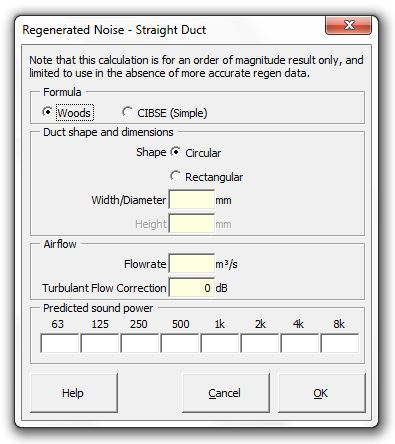### Strutt Help

Regenerated Noise - Straight Duct    1/1

Strutt|Mechanical Services|Regenerated Noise|Straight inserts sound power levels into the active row of the worksheet.The Woods method calculates the regenerated noise level using:

SWL = K + 10log_10 S + 55log_10 V - 45 dB

Where:
SWL is the total sound power level generated by the actual fitting (dB)
K = 38 dB
S is the actual duct area (m2)
V is the actual velocity (m/s)

Input required is the duct shape, duct dimensions and flow rate (m3/s)

Octave band sound power levels are calculated by applying the following corrections (Table 5.2, Woods):

 63 Hz 125 Hz 250 Hz 500 Hz 1 kHz 2 kHz 4 kHz 8 kHz -6 -6 -7 -7 -8 -10 -17 -29

The simple CIBSE method calculates the regenerated noise using:

L_W=K_t+10log(S)+60log(V)+C+Delta L_("turb")

where:
K_t is a constant depending on the duct fitting type. K_t=-10 for a straight duct
S is the duct cross-sectional area (mý)
V is the duct velocity (m/s)
C is a spectrum correction term (from Table 5.1 CIBSE Guide B)
DeltaL_(turb) is a user-inputted correction (dB) increasing the sound power levels to account for turbulent inflow onto the fittings.

Note there is a more-detailed CIBSE method based on the pressure loss factor etc, the Regenerated Noise from Duct Fittings (Detailed) calculation, which can be used if the pressure drop and loss factor of the fitting are known

References:

• Woods Practical Guide to Noise Control, p101.
• CIBSE Buide B(2005) Heating, ventilating, air conditioning and refrigeration Section 5.5.1